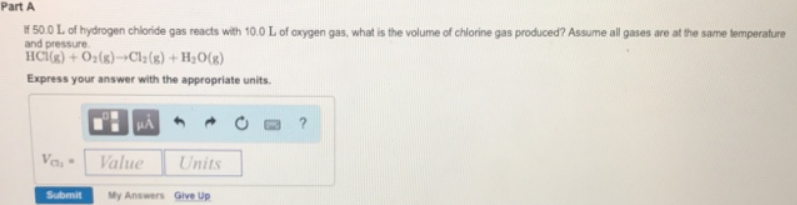# Problem: Part AIf 50.0 L of hydrogen chloride gas reacts with 10.0 L of oxygen gas, what is the volume of chlorine gas produced? Assume all gases are at the same temperature and pressure. HCl (g) + O2 (g) → Cl2 (g) + H2O (g) Express your answer with the appropriate units.

🤓 Based on our data, we think this question is relevant for Professor Veige's class at UF.

###### FREE Expert Solution###### Problem Details

Part A

If 50.0 L of hydrogen chloride gas reacts with 10.0 L of oxygen gas, what is the volume of chlorine gas produced? Assume all gases are at the same temperature and pressure.

HCl (g) + O2 (g) → Cl2 (g) + H2O (g)